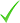HOME
• Find courses
• Free training quote
• Free Courses
• Online Courses
• Providers

# Machine Learning : Linear & Logistic Regression Online Course

Learning247
Course summary
Professional Training
27 GBP excl. VAT
Online courses
Course Dates
Online courses
27 GBP

## Course Description

This 'Linear & Logistic Regression' online training course will teach you how to build robust linear models and do logistic regressions in Excel, R, and Python that will stand up to scrutiny when you apply them to real world situations.

You'll learn about topics such as: understanding random variables, cause-effect relationships, maximum likelihood estimation, and so much more. Follow along with the experts as they break down these concepts in easy-to-understand lessons.

Course Highlights

Simple Regression :

• Method of least squares, Explaining variance, Forecasting an outcome
• Implement simple regression in Excel, R and Python
• Interpret regression results and avoid common pitfalls

Multiple Regression :

• Implement Multiple regression in Excel, R and Python
• Introduce a categorical variable

Logistic Regression :

• Applications of Logistic Regression, the link to Linear Regression and Machine Learning
• Solving logistic regression using Maximum Likelihood Estimation and Linear Regression
• Extending Binomial Logistic Regression to Multinomial Logistic Regression
• Implement Logistic regression to build a model stock price movements in Excel, R and Python

Course Requirements

• No statistics background required. Everything is built up from basic math
• The models are implemented in Excel, R and Python. Install these environments to follow along with the demos

Target Audience

• Data analysts who want to move from summarizing data to explaining and prediction
• Folks aspiring to be data scientists
• Any business professionals who want to apply Linear regression to solve relevant problems

Length: 5 hrs

## Course Outline

Chapter 01: Introduction

• Lesson 01: You, This Course, & Us!

Chapter 02: Connect the Dots with Linear Regression

• Lesson 01: Using Linear Regression to Connect the Dots
• Lesson 02: Two Common Applications of Regression
• Lesson 03: Extending Linear Regression to Fit Non-linear Relationships

Chapter 03: Basic Statistics Used for Regression

• Lesson 01: Understanding Mean & Variance
• Lesson 02: Understanding Random Variables
• Lesson 03: The Normal Distribution

Chapter 04: Simple Regression

• Lesson 01: Setting up a Regression Problem
• Lesson 02: Using Simple Regression to Explain Cause-Effect Relationships
• Lesson 03: Using Simple Regression for Explaining Variance
• Lesson 04: Using Simple Regression for Prediction
• Lesson 05: Interpreting the results of a Regression
• Lesson 06: Mitigating Risks in Simple Regression

Chapter 05: Applying Simple Regression

• Lesson 01: Applying Simple Regression in Excel
• Lesson 02: Applying Simple Regression in R
• Lesson 03: Applying Simple Regression in Python

Chapter 06: Multiple Regression

• Lesson 01: Introducing Multiple Regression
• Lesson 02: Some Risks inherent to Multiple Regression
• Lesson 03: Benefits of Multiple Regression
• Lesson 04: Introducing Categorical Variables
• Lesson 05: Interpreting Regression results “ Adjusted R-squared
• Lesson 06:  Interpreting Regression results “ Standard Errors of Coefficients
• Lesson 07: Interpreting Regression results “ t-statistics & p-values
• Lesson 08: Interpreting Regression results “ F-Statistic

Chapter 07: Applying Multiple Regression using Excel

• Lesson 01: Implementing Multiple Regression in Excel
• Lesson 02: Implementing Multiple Regression in R
• Lesson 03: Implementing Multiple Regression in Python

Chapter 08: Logistic Regression for Categorical Dependent Variables

• Lesson 01: Understanding the need for Logistic Regression
• Lesson 02: Setting up a Logistic Regression problem
• Lesson 03: Applications of Logistic Regression
• Lesson 04: The link between Linear & Logistic Regression
• Lesson 05: The link between Logistic Regression & Machine Learning

Chapter 09: Solving Logistic Regression

• Lesson 01: Understanding the intuition behind Logistic Regression & the S-curve
• Lesson 02: Solving Logistic Regression using Maximum Likelihood Estimation
• Lesson 03: Solving Logistic Regression using Linear Regression
• Lesson 04: Binomial vs Multinomial Logistic Regression

Chapter 10: Applying Logistic Regression

• Lesson 01: Predict Stock Price movements using Logistic Regression in Excel
• Lesson 02: Predict Stock Price movements using Logistic Regression in R
• Lesson 03: Predict Stock Price movements using Rule-based & Linear Regression
• Lesson 04: Predict Stock Price movements using Logistic Regression in Python
PACKAGE INCLUDES:

Length of Subscription: 12 Months Online On-Demand Access Running Time: 5 hrs Platform: Windows & MAC OS Level: Beginner to Advanced Project Files: Included

Learn anytime, anywhere, at home or on the go.

## Why choose Learning247

Gain a New Qualification - Get a verifiable qualification with Learning247 and improve your career prospects. Most of our courses CPD Accredited.

Wide Range of Courses - With over 800+ courses to choose from you're sure to find a course that interests you.

Study Anywhere & Any time - All of our online courses can be studied from your PC, MacBook , tablet or smart phone device.

## Learning247

As one of the market-leaders in distance-learning and self-study multimedia-based training, Learning247 provides the most cost-effective route to enable you to update and improve upon your skills; and all without the massive prices that are regularly seen throughout the training...

Read more and show all courses with this provider

## Request info

Fill out your details to find out more about Machine Learning : Linear & Logistic Regression Online Course.Contact the providerGet more informationRegister your interest

Country *

## Learning247

61 Caroline Street
B3 1UF Birmingham

Show phone number

Request informationRead the course summary on this pageFill in your details and Request informationReceive all the info you need